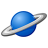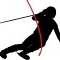QlikView App Dev

Discussion Board for collaboration related to QlikView App Development.

Announcements
QlikWorld 2022, LIVE in Denver CO., May 16-19, 2022. REGISTER NOW TO RECEIVE EARLY BIRD PRICING
cancel
Showing results for
Did you mean:Specialist III

Want to calculate market share based on the partial sums(second dimension percentages)

I have a pivot table where I have an expression market share which is calculated by the following expression

Sum({1<Commodity=p(Commodity),QuarterYear={\$(vCurrentQuarter)}>}Spend)

/

Sum( total{1<Commodity=p(Commodity),QuarterYear={\$(vCurrentQuarter)}>}Spend)

Here the problem is I want the denominator value as the partial sum of that particular dimension value but because I have taken

total qualifier it is taking the sum of all dimension values.

for eg , for commodity ABC,Supplier XXP the spend is 82,916, the market share should be 53.177(82916.90/155924.04)

but I am getting 2.44 because I have used total qualifier it is taking the whole commodities spend.

How to get that partial sum of commodity in the expression.

1 Solution

Accepted SolutionsSpecialist III
Author

Finally got it , when we have calculated dimension we have to use advanced aggregation using aggr().

The following post helped me alot

Quick Development Tips: Qlikview: Aggregation 1: How to calculate percentages at second dimension le...

The expression which I have used for market share is

Sum({1<Commodity=p(Commodity),QuarterYear={\$(vCurrentQuarter)}>}Spend)

/

Aggr(NODISTINCT Sum({1<Commodity=p(Commodity),QuarterYear={\$(vCurrentQuarter)}>}Spend),Commodity)

Thanks

Kiran Kumar .CH

12 RepliesMVP

Sum({1<Commodity=p(Commodity),QuarterYear={\$(vCurrentQuarter)}>}Spend)

/

Sum( total <Commodity>{1<Commodity=p(Commodity),QuarterYear={\$(vCurrentQuarter)}>}Spend)Partner

Hi,

Try to use this expression

Sum({1<Commodity=p(Commodity),QuarterYear={\$(vCurrentQuarter)}>}Spend)

/

Sum( total <Commodity> {1<Commodity=p(Commodity),QuarterYear={\$(vCurrentQuarter)}>}Spend)

Regards,

Sergey

Regards,
SergeySpecialist

Kiran,

Try the expression below to reachyour requirement and also to get the % in Total rows (total commodity / total spent):

=if(Dimensionality()=2,

Sum({1<Commodity=p(Commodity),QuarterYear={\$(vCurrentQuarter)}>}Spend)

/Sum({1<Commodity=p(Commodity),QuarterYear={\$(vCurrentQuarter)}>} TOTAL <Commodity> Spend),

if(Dimensionality()=1,

Sum({1<Commodity=p(Commodity),QuarterYear={\$(vCurrentQuarter)}>}Spend)

/Sum({1<Commodity=p(Commodity),QuarterYear={\$(vCurrentQuarter)}>} TOTAL Spend)))

Marc.Specialist III
Author

No ,

I didnt get it, every market share converted to 100% , even I tried with <commodity> and <supplier>

Any more suggestions ??MVP

Hi,

Try this expression you need to use Commodity in Total like below

Sum({1<Commodity=p(Commodity),QuarterYear={\$(vCurrentQuarter)}>}Spend)

/

Sum({1<Commodity=p(Commodity),QuarterYear={\$(vCurrentQuarter)}>} TOTAL <Commodity> Spend)

TOTAL <Commodity> - This will ignore the Commodity dimension and you will get the Commodity wise total.

Hope this helps you.

Regards,

Jagan.MVP

Sum({<Commodity=p(Commodity),QuarterYear={\$(vCurrentQuarter)}>}Spend)

/

Sum( total <Commodity>{<Commodity=p(Commodity),QuarterYear={\$(vCurrentQuarter)}>}Spend)Specialist

Kiran,

Use my expression posted before in order to avoid 100% values.

Marc.Specialist III
Author

Hi Marc,

All my market share values turned to 100% except the partial sums.

Thank You

KiranMVP

Can you provide sample data or sample apps.....Community Browser